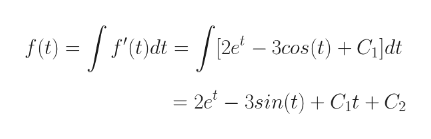# Find f iff"(t) 2e 3 sin(t), f(0)= 5, f(n) 2f(t)=

Question
7 viewshelp_outlineImage TranscriptioncloseFind f iff"(t) 2e 3 sin(t), f(0)= 5, f(n) 2 f(t) = fullscreen
check_circle

Step 1

Please see the white board. C1 is the constant of integration.

Step 2

Please see the white board. C2 is the constant of integration.help_outlineImage Transcriptionclosef (t) f'Cdt 3cos (t)Cldt [2e = 2e - 3sin(t) + C\t + C2 fullscreen
Step 3

f(t) = 2et - 3sin(t) + C1t + C2

f(0) = 2 + C2 = 5 Hence, C2 = 5 - 2 = 3

f(π) =2eπ -3sin(π) + C1π + C2 = 2eπ + C1π + 3 = 2

Hence, C1 = (2 &ndash...

### Want to see the full answer?

See Solution

#### Want to see this answer and more?

Solutions are written by subject experts who are available 24/7. Questions are typically answered within 1 hour.*

See Solution
*Response times may vary by subject and question.
Tagged in

### Calculus